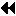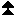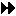# 6.5. ExamplesUp: One-Sided Communications Next: Error Handling Previous: Miscellaneous Clarifications

Example The following example shows a generic loosely synchronous, iterative code, using fence synchronization. The window at each process consists of array A, which contains the origin and target buffers of the put calls.

```...
while(!converged(A)){
update(A);
MPI_Win_fence(MPI_MODE_NOPRECEDE, win);
for(i=0; i < toneighbors; i++)
MPI_Put(&frombuf[i], 1, fromtype[i], toneighbor[i],
todisp[i], 1, totype[i], win);
MPI_Win_fence((MPI_MODE_NOSTORE | MPI_MODE_NOSUCCEED), win);
}
```
The same code could be written with get, rather than put. Note that, during the communication phase, each window is concurrently read (as origin buffer of puts) and written (as target buffer of puts). This is OK, provided that there is no overlap between the target buffer of a put and another communication buffer.

Example Same generic example, with more computation/communication overlap. We assume that the update phase is broken in two subphases: the first, where the ``boundary,'' which is involved in communication, is updated, and the second, where the ``core,'' which neither use nor provide communicated data, is updated.

```...
while(!converged(A)){
update_boundary(A);
MPI_Win_fence((MPI_MODE_NOPUT | MPI_MODE_NOPRECEDE), win);
for(i=0; i < fromneighbors; i++)
MPI_Get(&tobuf[i], 1, totype[i], fromneighbor[i],
fromdisp[i], 1, fromtype[i], win);
update_core(A);
MPI_Win_fence(MPI_MODE_NOSUCCEED, win);
}
```
The get communication can be concurrent with the core update, since they do not access the same locations, and the local update of the origin buffer by the get call can be concurrent with the local update of the core by the update_core call. In order to get similar overlap with put communication we would need to use separate windows for the core and for the boundary. This is required because we do not allow local stores to be concurrent with puts on the same, or on overlapping, windows.

Example Same code as in Example Examples , rewritten using post-start-complete-wait.

```...
while(!converged(A)){
update(A);
MPI_Win_post(fromgroup, 0, win);
MPI_Win_start(togroup, 0, win);
for(i=0; i < toneighbors; i++)
MPI_Put(&frombuf[i], 1, fromtype[i], toneighbor[i],
todisp[i], 1, totype[i], win);
MPI_Win_complete(win);
MPI_Win_wait(win);
}
```

Example Same example, with split phases, as in Example Examples .

```...
while(!converged(A)){
update_boundary(A);
MPI_Win_post(togroup, MPI_MODE_NOPUT, win);
MPI_Win_start(fromgroup, 0, win);
for(i=0; i < fromneighbors; i++)
MPI_Get(&tobuf[i], 1, totype[i], fromneighbor[i],
fromdisp[i], 1, fromtype[i], win);
update_core(A);
MPI_Win_complete(win);
MPI_Win_wait(win);
}
```

Example A checkerboard, or double buffer communication pattern, that allows more computation/communication overlap. Array A0 is updated using values of array A1, and vice versa. We assume that communication is symmetric: if process A gets data from process B, then process B gets data from process A. Window wini consists of array Ai.

```...
if (!converged(A0,A1))
MPI_Win_post(neighbors, (MPI_MODE_NOCHECK | MPI_MODE_NOPUT), win0);
MPI_Barrier(comm0);
/* the barrier is needed because the start call inside the
loop uses the nocheck option */
while(!converged(A0, A1)){
/* communication on A0 and computation on A1 */
update2(A1, A0); /* local update of A1 that depends on A0 (and A1) */
MPI_Win_start(neighbors, MPI_MODE_NOCHECK, win0);
for(i=0; i < neighbors; i++)
MPI_Get(&tobuf0[i], 1, totype0[i], neighbor[i],
fromdisp0[i], 1, fromtype0[i], win0);
update1(A1); /* local update of A1 that is
concurrent with communication that updates A0 */
MPI_Win_post(neighbors, (MPI_MODE_NOCHECK | MPI_MODE_NOPUT), win1);
MPI_Win_complete(win0);
MPI_Win_wait(win0);

/* communication on A1 and computation on A0 */
update2(A0, A1); /* local update of A0 that depends on A1 (and A0)*/
MPI_Win_start(neighbors, MPI_MODE_NOCHECK, win1);
for(i=0; i < neighbors; i++)
MPI_Get(&tobuf1[i], 1, totype1[i], neighbor[i],
fromdisp1[i], 1, fromtype1[i], win1);
update1(A0); /* local update of A0 that depends on A0 only,
concurrent with communication that updates A1 */
if (!converged(A0,A1))
MPI_Win_post(neighbors, (MPI_MODE_NOCHECK | MPI_MODE_NOPUT), win0);
MPI_Win_complete(win1);
MPI_Win_wait(win1);
}
```
A process posts the local window associated with win0 before it completes RMA accesses to the remote windows associated with win1. When the wait(win1) call returns, then all neighbors of the calling process have posted the windows associated with win0. Conversely, when the wait(win0) call returns, then all neighbors of the calling process have posted the windows associated with win1. Therefore, the nocheck option can be used with the calls to MPI_WIN_START.

Put calls can be used, instead of get calls, if the area of array A0 (resp. A1) used by the update(A1, A0) (resp. update(A0, A1)) call is disjoint from the area modified by the RMA communication. On some systems, a put call may be more efficient than a get call, as it requires information exchange only in one direction.Up: One-Sided Communications Next: Error Handling Previous: Miscellaneous Clarifications